SSC BOARD PAPERS IMPORTANT TOPICS COVERED FOR BOARD EXAM 2024

# Measurement Of Matter

##### Class 9th Science & Technology MHB Solution
Exercises
2. Write symbols of the following elements and the radicals obtained from them, and indicate…
3. Sodium sulphate Write the steps in deducing the chemical formulae of the following…
4. potassium nitrate Write the steps in deducing the chemical formulae of the following…
5. Ferric phosphate Write the steps in deducing the chemical formulae of the following…
6. calcium oxide Write the steps in deducing the chemical formulae of the following…
7. Aluminium hydroxide Write the steps in deducing the chemical formulae of the following…
8. Explain how the element sodium is monovalent. Write answers to the following questions and…
9. M is a bivalent metal. Write down the steps to find the chemical formulae of its compounds…
10. Explain the need for a reference atom for atomic mass. Give some information about two…
11. What is meant by Unified Atomic Mass.' Write answers to the following questions and…
12. Explain with examples what is meant by a 'mole' of a substance. Write answers to the…
13. Write the names of the following compounds and deduce their molecular masses. Na2SO4,…
14. Two samples ‘m’ and ‘n’ of slaked lime were obtained from two different reactions. The…
15. Deduce the number of molecules of the following compounds in the given quantities. 32g…
16. If 0.2 mol of the following substances are required how many grams of those substances…

###### Exercises
Question 1.

Give examples.

D. Metals with variable valency

Positively charged ions are called cations.

Examples:- NH4+ ,K + ,Fe + 2,Al + 3

The cationic radicals are called basic radicals. Basic radicals are formed by removal of electrons from the atoms of metals.

Example: Na + , Cu2 + ,Ag + ,Mg + 2,Au + 3

When a radical is a group of atoms carrying a charge, it is called composite radical.

Examples:-

NO3-, ClO3- , SO42-, NH4+ , MnO4-

D. Metals with variable valency

Under different conditions, the atoms of some elements give away or take up different numbers of electrons. In such cases, those elements exhibit more than one valency. This property of elements is called variable valency.

Examples:-

Iron (Fe) exhibits the variable valencies 2 and 3: Fe2 + and Fe3 +

Gold(Au) exhibits the variable valencies 1 and 3: Au + and Au3 +

Copper(Cu) exhibits the variable valencies 1 and 2: Cu + and Cu2 +

Mercury(Hg) exhibits the variable valencies 1 and 2: Hg + and Hg2 +

Bivalent refers to an element whose atom can replace 2 atoms of hydrogen or another univalent element. It also refers to a radical that has the same valence as a bivalent atom.

Examples: SO42-, CO32-, C2O42-

A trivalent basic radical is a cation that has a valency of three. Examples of trivalence basic radicals are Al3 + ,Cr3 + , Bi3 + and Fe + 2

Question 2.

Write symbols of the following elements and the radicals obtained from them, and indicate the charge on the radicals.

Mercury, potassium, nitrogen, copper, sulphur, carbon, chlorine, oxygen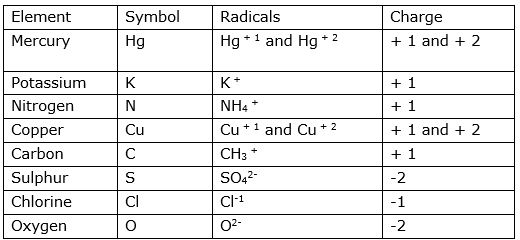Question 3.

Write the steps in deducing the chemical formulae of the following compounds.

Sodium sulphate

Sodium Sulphate-

Formula: Na2SO4

Steps to obtain the chemical formula:

Step 1: To write the symbols of the radicals. (Basic radical on the left.)

Na SO4

Step 2: To write the valency below the respective radical. Na SO4

1 2

Step 3: To cross-multiply as shown by the arrows the number of the radicals.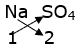Step 4: To write down the chemical formula of the compound.

Na2SO4

Question 4.

Write the steps in deducing the chemical formulae of the following compounds.

potassium nitrate

Potassium Nitrate-

Formula: KNO3

Step 1: To write the symbols of the radicals. (Basic radical on the left.)

K NO3

Step 2: To write the valency below the respective radical. K NO3

1 1

Step 3: To cross-multiply as shown by the arrows the number of the radicals.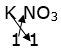Step 4: To write down the chemical formula of the compound.

KNO3

Question 5.

Write the steps in deducing the chemical formulae of the following compounds.

Ferric phosphate

Ferric Phosphate-

Formula:- FePO4

Step 1: To write the symbols of the radicals.(Basic radical on the left.)

Fe PO4

Step 2: To write the valency below the respective radical. Fe PO4

3 3

Step 3: To cross-multiply as shown by the arrows the number of the radicals.

Fe PO4

3 3

Step4: To write down the chemical formula of the compound.

FePO4

Question 6.

Write the steps in deducing the chemical formulae of the following compounds.

calcium oxide

Calcium Oxide-

Formula:- CaO

Step 1 :

To write the symbols of the radicals. (Basic radical on the left.)

Ca O

Step 2: To write the valency below the respective radical.

Ca O

+ 2 -2

Step 3: To cross-multiply as shown by the arrows the number of the radicals.

Ca O

+ 2 -2

Step4: To write down the chemical formula of the compound in the simplest form.

CaO

Question 7.

Write the steps in deducing the chemical formulae of the following compounds.

Aluminium hydroxide

Aluminum Hydroxide-

Formula: -Al(OH)3

Step 1:

To write the symbols of the radicals. (Basic radical on the left.)

Al OH

Step 2: To write the valency below the respective radical.

Al OH

3 1

Step 3: To cross-multiply as shown by the arrows the number of the radicals.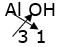Step 4 To write down the chemical formula of the compound. Al(OH)3

Question 8.

Explain how the element sodium is monovalent.

A. The atomic number of Sodium is 11. Sodium has 1 electron in its outermost shell and to acquire noble gas configuration it will lose one electron or it needs to gain 7 electrons. However, the former is possible. Hence it will loose 1 electron and so the element sodium is monovalent.

Question 9.

M is a bivalent metal. Write down the steps to find the chemical formulae of its compounds formed with the radicals, sulphate and phosphate

M is bivalent which means it has a valency of 2.

(i)For sulphate

Step 1: To write the symbols of the radicals. (Basic radical on the left.)

M SO4

Step 2: To write the valency below the respective radical.

M SO4

+ 2 -2

Step 3: To cross-multiply as shown by the arrows the number of the radicals.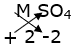Step4: To write down the chemical formula of the compound in the simplest form.

MSO4

(ii) for phosphate

Step 1:To write the symbols of the radicals. (Basic radical on the left.)

M PO4

Step 2: To write the valency below the respective radical.

M PO4

+ 2 -3

Step 3: To cross-multiply as shown by the arrows the number of the radicals.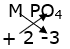Step4: To write down the chemical formula of the compound in the simplest form.

M3(PO4)2

Question 10.

Explain the need for a reference atom for atomic mass. Give some information about two reference atoms.

It was not possible for scientists of the 19th century to measure atomic mass accurately. Therefore, the concept of ‘relative mass of an atom’ was put forth. A reference atom was required for expressing the relative mass of an atom. The hydrogen atom is the lightest was initially chosen as the reference atom. The relative mass of a hydrogen atom which has only one proton in its nucleus was accepted as one (1).

Therefore, the magnitude of the relative atomic masses of various atoms became equal to their atomic mass number (p + n).

In the course of time, different atoms were chosen as reference

atoms. Finally in 1961, the carbon atom was selected as the reference atom. In this scale, the relative mass a carbon atom was accepted as 12. The relative atomic mass of one hydrogen

atom compared to the carbon atom becomes 12 x 1/12, that is 1. The mass of one proton and of one neutron on the scale of relative atomic masses is approximately one.

Question 11.

What is meant by Unified Atomic Mass.'

The unified atomic mass unit or Dalton is a standard unit of mass that quantifies mass on an atomic or molecular scale. One unified atomic mass unit is approximately the mass of one nucleon and is numerically equivalent to 1 g/mol.

Question 12.

Explain with examples what is meant by a 'mole' of a substance.

1 mole of a compound is the mass of that substance in grams equal in magnitude to its molecular mass. The SI unit is mol.

Number of moles of a substance (n) =

(Mass of substance in grams)/(Molecular mass of substance)

the molecular mass of oxygen is 32u, and therefore 32g oxygen is 1mole of oxygen. The molecular mass of water is 18u. Therefore, 18g of water make 1 mole of water.

Question 13.

Write the names of the following compounds and deduce their molecular masses.

Na2SO4, K2CO3, CO2, NaOH, MgCl2, AlPO4, NaHCO3

i. Na2SO4 : Sodium Sulphate

Steps for finding the molecular mass:

Molecular mass of Na2SO4 = 2(Atomic mass of Na) + 1(Atomic mass of S) + 4(Atomic mass of O)

⇒ Molecular Mass = 2(23) + 1(32) + 4(16)

= 46 + 32 + 64

= 142 g/mol

ii.K2CO3: Potassium Carbonate

Steps for finding the molecular mass:

Molecular mass of K2CO3 = 2× (Atomic mass of K) + 1× (Atomic mass of C) + 3× (Atomic mass of O)

Molecular Mass = 2× (39) + 1× (12) + 3× (16)

= 78 + 12 + 48

= 138 g/mol

iii.CO2:- Carbondioxide

Molecular mass of CO2 = 1× (Atomic mass of C) + 2× (Atomic mass of O)

Molecular Mass = 1× (12) + 2× (16)

= 12 + 32

= 44 g/mol

iv.NaOH:Sodium Hydroxide

Molecular mass of NaOH = 1× (Atomic mass of Na) + 1× (Atomic mass of O) + 1× (Atomic mass of H)

Molecular Mass = 1× (23) + 1× (16) + 1× (1)

= 23 + 16 + 1

= 40 g/mol

v.MgCl2:Magnesium Chloride

Molecular mass of MgCl2 = 1× (Atomic mass of Mg) + 2× (Atomic mass of Cl)

Molecular Mass = 1× (24) + 2× (35.5)

= 24 + 70

= 94 g/mol

vi.AlPO4:-Aluminium Phosphate

Molecular mass of AlPO4 = 1× (Atomic mass of Al) + 1× (Atomic mass of P) + 4× (Atomic mass of O)

Molecular Mass = 1× (27) + 1× (31) + 4× (16)

= 27 + 31 + 64

= 122 g/mol

vii.NaHCO3: Sodium Bicarbonate

Molecular mass of NaHCO3 = 1× (Atomic mass of Na) + 1× (Atomic mass of H) + 1× (Atomic mass of C) + 3× (Atomic mass of O)

Molecular Mass = 1× (23) + 1× (1) + 1× (12) + 3× (16)

= 23 + 1 + 12 + 48

= 84 g/mol

Question 14.

Two samples ‘m’ and ‘n’ of slaked lime were obtained from two different reactions. The details about their composition are as follows:

‘sample m’ mass: 7g

Mass of constituent oxygen: 2g

Mass of constituent calcium: 5g

‘sample n’ mass: 1.4g

Mass of constituent oxygen: 0.4g

Mass of constituent calcium: 1.0g

Which law of chemical combination does this prove? Explain.

Slaked lime = calcium hydroxide {Ca(OH)2}

The law of constant proportions states that “The proportion by weight of the constituent elements in the various samples of a compound is fixed,”

The proportion by weight of oxygen and calcium in slaked lime(in sample m) is 2:5 i.e. 0.4:1

The proportion by weight of oxygen and calcium in slaked lime(in sample n) is 0.4:1.0

Since the proportions of constituent elements remain fixed it follows the law of constant proportion.

Question 15.

Deduce the number of molecules of the following compounds in the given quantities.

32g oxygen, 90g water, 8.8g carbon dioxide, 7.1g chlorine.

(a) 32g of oxygen

Moles = Mass of substance in grams/Molecular mass

Molecular mass of oxygen = 16

Mass of a substance = 32g

Moles(n) = 32/16 = 2

n = 2 mol

2 mol of oxygen contains 6.022 x 1023 molecules.

2 mol oxygen contains 2x6.022x1023 molecules = 12.046 x 1023 molecules.

(b) 90g water

Moles = Mass of substance in grams/Molecular mass

Molecular mass of water = 18

Mass of a substance = 90g

Moles(n) = 90/18 = 5

n = 5 mol

5 mol of oxygen contains 6.022 x 1023 molecules.

5 mol oxygen contains 5x6.022x1023 molecules = 30.115× 1023 molecules.

(c) 8.8g carbon dioxide

Moles = Mass of substance in grams/Molecular mass

Molecular mass of CO2 = 44

Mass of a substance = 8.8g

Moles(n) = 8.8/44 = 0.2

n = 0.2 mol

0.2 mol of oxygen contains 6.022 x 1023 molecules.

0.2 mol oxygen contains 0.2x6.022x1023 molecules = 1.2046 x 1023 molecules.

(d) 7.1g chlorine.

Moles = Mass of substance in grams/Molecular mass

Molecular mass of chlorine = 35.5

Mass of a substance = 7.1g

Moles(n) = 7.1/35.5 = 0.2

n = 0.2 mol

0.2 mol of oxygen contains 6.022 x 1023 molecules.

0.2 mol oxygen contains 0.2x6.022x1023 molecules = 1.2046 x 1023 molecules.

Question 16.

If 0.2 mol of the following substances are required how many grams of those substances should be taken?

Sodium chloride, magnesium oxide,calcium carbonate

(a) Sodium Chloride

Moles = Mass of substance in grams /Molecular Mass(in g/mol)

Molecular mass of NaCl = 1× (Atomic mass of Na) + 1× (Atomics mass of Cl)

= 1× (23) + 1× (35.5)

= 55.5

Mole = 0.2 mol(given)

0.2 = given mass/55.5

Given mass = 0.2× 55.5

= 11.1g

(b) Magnesium Oxide

Moles = Mass of substance in grams /Molecular Mass(in g/mol)

Molecular mass of MgO = 1× (Atomic mass of Mg) + 1× (Atomics mass of O)

= 1× (24) + 1× (16)

= 40

Mole = 0.2 mol(given)

0.2 = given mass/40

Given mass = 0.2× 40

= 8g

(c) Calcium Carbonate

Moles = Mass of substance in grams /Molecular Mass(in g/mol)

Molecular mass of CaCO3 = 1× (Atomic mass of Ca) + 1× (Atomics mass of C) + 3× (atomic mass of O)

= 1× (40) + 1× (12) + 3× (16)

= 100

Mole = 0.2 mol(given)

0.2 = given mass/100

Given mass = 0.2× 100

= 20g